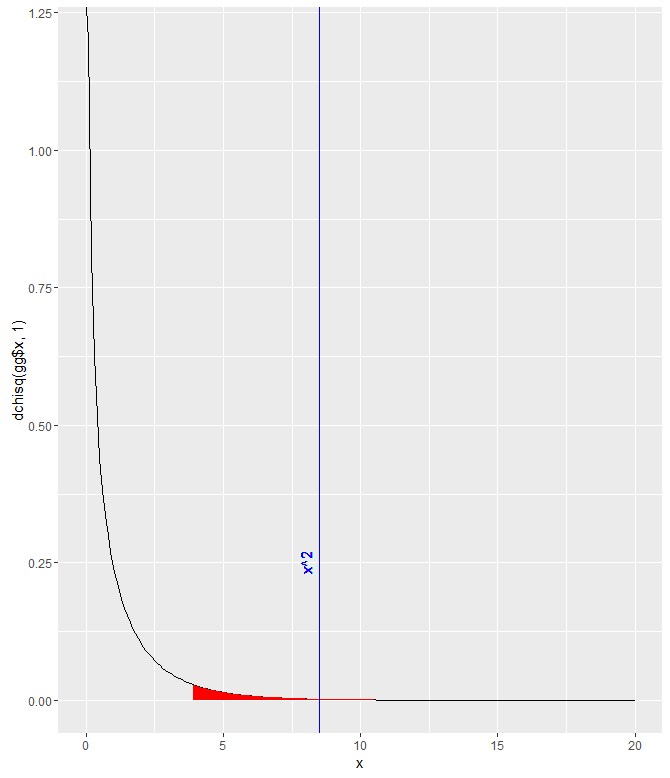Select Page

## Chi-Squared testing

Null hypothesis:  The proportion of people who choose the same hotel again will be the same for beach combers and windsurfers.

To start off, I constructed my data.frame in a very roundabout manner:

```>Beachcomber <- c(163,64,227)
>Windsurfer <- c(154,108,262)
>Choose_again <- c("Yes", "No", "Total")
>dat <- data.frame(Choose_again, Beachcomber, Windsurfer)
>dat\$Total <- dat\$Beachcomber + dat\$Windsurfer
>dat <- dat[,-1]
>rownames(dat) <- c("Yes", "no","total")

>dat

Beachcomber Windsurfer Total
Yes           163        154   317
no             64        108   172
total         227        262   489```

Next, I run the chi-test and save it in an object:

```>res<-chisq.test(dat[1:2,1:2])
> res

Pearson's Chi-squared test with Yates' continuity correction

data: dat[1:2, 1:2]
X-squared = 8.4903, df = 1, p-value = 0.0035```

I was stuck on that for a while, trying to figure out exactly which parts of the data.frame needed to be included/excluded.  I did notice that this way of doing it didn’t give me the error that other combinations did though.  I assume it’s because the function implemented Yates’ continuity correction, which is automatically implemented for 2×2 tables.  The p-value is quite small, so at a threshold of p=.05, the null hypothesis would be rejected and we’d say that it appears that the proportion of people who choose to return to their hotel is different for each group.```gg <- data.frame(x = seq(0,20,.1))
gg\$y <- dchisq(gg\$x, 1)
ggplot(gg) +
geom_path(aes(x,y)) +
geom_ribbon(data=gg[gg\$x>qchisq(.05,1,lower.tail=FALSE),], aes(x,ymin=0, ymax=y), fill="red")+
geom_vline(xintercept = res\$statistic, color = "blue")+
labs( x = "x", y = "dchisq(gg\$x, 1)")+
geom_text(aes(x=8, label="x^2", y=0.25), colour="blue", angle=90)```